# 什么是 KMP 算法（详解）KMP 是三位大牛：D.E.Knuth、J.H.Morris 和 V.R.Pratt 同时发现的。其中第一位就是《计算机程序设计艺术》的作者！！

KMP 算法要解决的问题就是在字符串（也叫主串）中的模式（pattern）定位问题。说简单点就是我们平时常说的关键字搜索。模式串就是关键字（接下来称它为 P），如果它在一个主串（接下来称为 T）中出现，就返回它的具体位置，否则返回-1（常用手段）。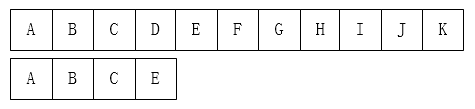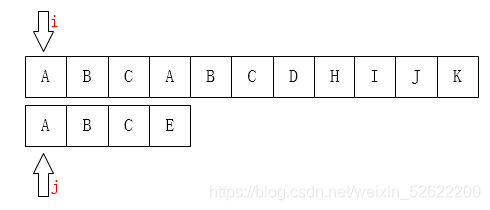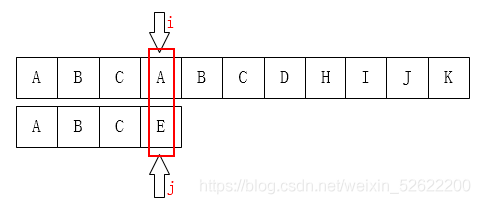A 和 E 不相等，那就把 i 指针移回第 1 位（假设下标从 0 开始），j 移动到模式串的第 0 位，然后又重新开始这个步骤：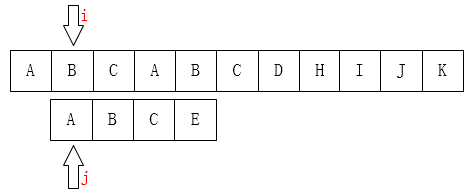/** * 暴力破解法 * @param ts 主串 * @param ps 模式串 * @return 如果找到，返回在主串中第一个字符出现的下标，否则为-1 */public static int bf(String ts, String ps) {    char[] t = ts.toCharArray();    char[] p = ps.toCharArray();    int i = 0; // 主串的位置    int j = 0; // 模式串的位置    while (i < t.length && j < p.length) {       if (t[i] == p[j]) { // 当两个字符相同，就比较下一个           i++;           j++;       } else {           i = i - j + 1; // 一旦不匹配，i后退           j = 0; // j归0       }    }    if (j == p.length) {       return i - j;    } else {       return -1;    }}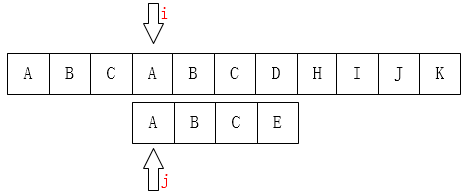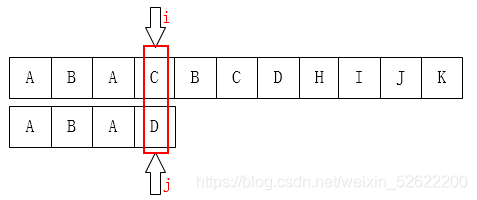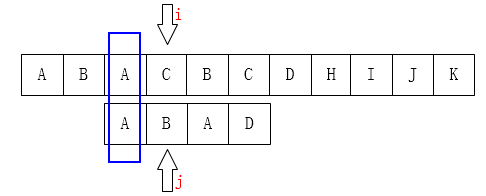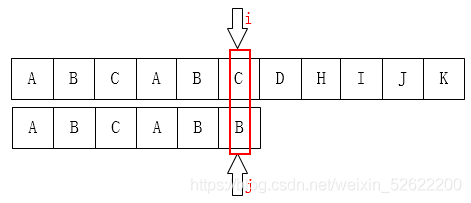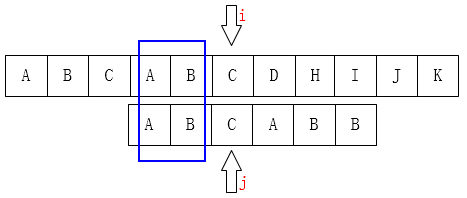P[0 ~ k-1] == P[j-k ~ j-1]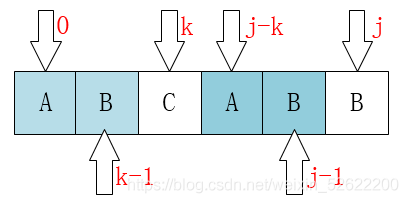当T[i] != P[j]时有T[i-j ~ i-1] == P[0 ~ j-1]由P[0 ~ k-1] == P[j-k ~ j-1]必然：T[i-k ~ i-1] == P[0 ~ k-1]public static int[] getNext(String ps) {    char[] p = ps.toCharArray();    int[] next = new int[p.length];    next = -1;    int j = 0;    int k = -1;    while (j < p.length - 1) {       if (k == -1 || p[j] == p[k]) {           next[++j] = ++k;       } else {           k = next[k];       }    }    return next;}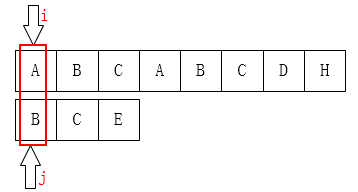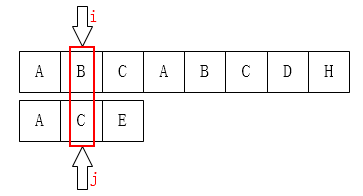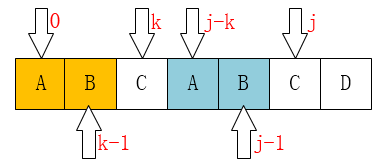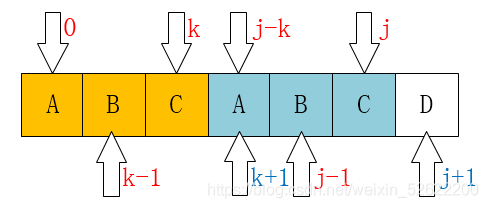当P[k] == P[j]时，有next[j+1] == next[j] + 1

因为在P[j]之前已经有P[0 ~ k-1] == p[j-k ~ j-1]。（next[j] == k）这时候现有P[k] == P[j]，我们是不是可以得到P[0 ~ k-1] + P[k] == p[j-k ~ j-1] + P[j]。即：P[0 ~ k] == P[j-k ~ j]，即next[j+1] == k + 1 == next[j] + 1。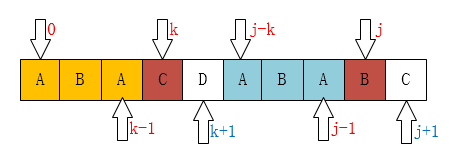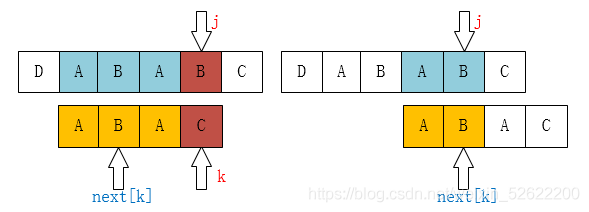public static int KMP(String ts, String ps) {    char[] t = ts.toCharArray();    char[] p = ps.toCharArray();    int i = 0; // 主串的位置    int j = 0; // 模式串的位置    int[] next = getNext(ps);    while (i < t.length && j < p.length) {       if (j == -1 || t[i] == p[j]) { // 当j为-1时，要移动的是i，当然j也要归0           i++;           j++;       } else {           // i不需要回溯了           // i = i - j + 1;           j = next[j]; // j回到指定位置       }    }    if (j == p.length) {       return i - j;    } else {       return -1;    }}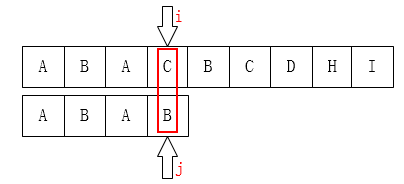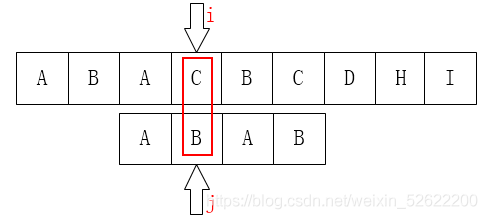public static int[] getNext(String ps) {    char[] p = ps.toCharArray();    int[] next = new int[p.length];    next = -1;    int j = 0;    int k = -1;    while (j < p.length - 1) {       if (k == -1 || p[j] == p[k]) {           if (p[++j] == p[++k]) { // 当两个字符相等时要跳过              next[j] = next[k];           } else {              next[j] = k;           }       } else {           k = next[k];       }    }    return next;}## 评论